Next: Low-Frequency Wave Propagation Up: Waves in Cold Plasmas Previous: Cutoff and Resonance

# Waves in an Unmagnetized Plasma

Let us now investigate the cold-plasma dispersion relation in detail. It is instructive to first consider the limit in which the equilibrium magnetic field goes to zero. In the absence of the magnetic field, there is no preferred direction, so we can, without loss of generality, assume that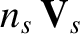is directed along the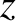-axis (i.e.,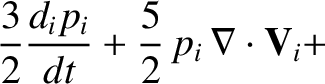). In the zero magnetic field limit (i.e.,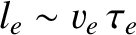), the eigenmode equation (490) reduces to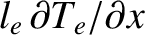(516)

where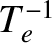(517)

Here, we have neglected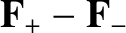with respect to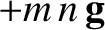.

It is clear from Eq. (516) that there are two types of wave. The first possesses the eigenvector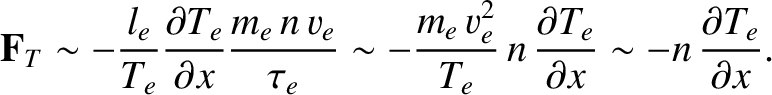, and has the dispersion relation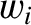(518)

The second possesses the eigenvector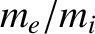, and has the dispersion relation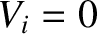(519)

Here,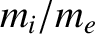,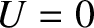, and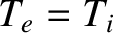are arbitrary non-zero quantities.

The first wave hasparallel to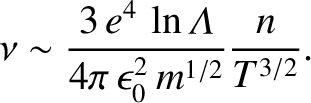, and is, thus, a longitudinal wave. This wave is know as the plasma wave, and possesses the fixed frequency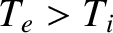. Note that ifis parallel tothen it follows from Eq. (454) that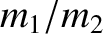. In other words, the wave is purely electrostatic in nature. In fact, a plasma wave is an electrostatic oscillation of the type discussed in Sect. 1.5. Since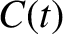is independent of, the group velocity,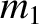(520)

associated with a plasma wave, is zero. As we shall demonstrate later on, the group velocity is the propagation velocity of localized wave packets. It is clear that the plasma wave is not a propagating wave, but instead has the property than an oscillation set up in one region of the plasma remains localized in that region. It should be noted, however, that in a warm'' plasma (i.e., a plasma with a finite thermal velocity) the plasma wave acquires a non-zero, albeit very small, group velocity (see Sect. 6.2).

The second wave is a transverse wave, withperpendicular to. There are two independent linear polarizations of this wave, which propagate at identical velocities, just like a vacuum electromagnetic wave. The dispersion relation (519) can be rearranged to give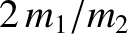(521)

showing that this wave is just the conventional electromagnetic wave, whose vacuum dispersion relation is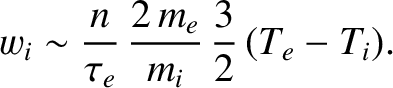, modified by the presence of the plasma. An important property, which follows immediately from the above expression, is that for the propagation of this wave we need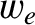. Sinceis proportional to the square root of the plasma density, it follows that electromagnetic radiation of a given frequency will only propagate through a plasma when the plasma density falls below a critical value.Next: Low-Frequency Wave Propagation Up: Waves in Cold Plasmas Previous: Cutoff and Resonance
Richard Fitzpatrick 2011-03-31